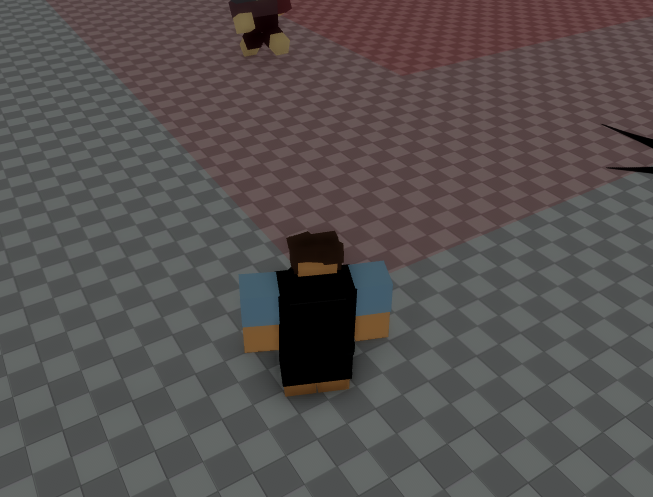# Help with Magnitude (the size of the area is 2x of the original)

I am trying to get the distance of the player from a centered part:

``````local distance = (pos - chr.HumanoidRootPart.Position).Magnitude
print(math.floor(distance))
``````

The centered red part is (25,1,25)
and the outer part is (50,1,50)
When I print the values of the player distance from the centered red part I get

` ▶ 25 (x14) - NewSystem:32`
I am standing outside the (50,1,50) part, and when I stand near the inner one I get printed 12(.5) which is half the value.
Anyone has an idea on how to fix this and on how to make it so I can get the distance of 25 from the corners as well? (I mean not 25, but 12.5 because it’s the corners)This is because the `Position` property of a BasePart instance describes its center point in the world space.

How can I get the center point of it like I intend to do?

25 is the correct value because the size is 50 from 1 side all the way to the other side but is 25 from the centre

if you want to measure in a square shape then you cant use Magnitude as that will give you a circle shape

this is how you can measure in a square shape

``````local characterPosition = character.HumanoidRootPart.Position
local partPosition = part.Position
local xDistance = math.abs(partPosition.X - characterPosition.X)
local zDistance = math.abs(partPosition.Z - characterPosition.Z)
local distance = math.max(xDistance, zDistance)
print(distance)
``````
1 Like